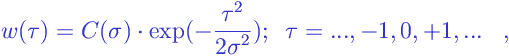# Gaussian Filter

Gaussian Filter:

The Gaussian filter is a linear filter that is usually used as a smoother . The output of the gaussian filter at the momentis the weighted mean of the input values, and the weights are defined by formulawhere

•is the “distance” in time from the current moment;
•is the parameter of the Gaussian filter;
•is the normalization constant chosen to make the sum of all weights equal to the unit value.

If you plot the values ofagainst, then the plot coincides with the famous bell-like curve describing the density of the gaussian distribution . This explains the word “gaussian” in the name of the filter.

The gaussian filter is completely defined by a single parameter. The greater the value of, the wider the window function, and, hence, the greater the degree of smoothing.

The gaussian filter provides better suppression of higher frequencies than the rectangular filter and the triangular filter .

Besides the one-dimensional gaussian filter described above, there are extensions to the case of two dimensions, say,. Such two-dimensional gaussian filters are widely used in image processing .

Browse Other Glossary Entries

## Test Yourself

Planning on taking an introductory statistics course, but not sure if you need to start at the beginning? Review the course description for each of our introductory statistics courses and estimate which best matches your level, then take the self test for that course. If you get all or almost all the questions correct, move on and take the next test.

### Data Analytics

Considering becoming adata scientist, customer analyst or our data science certificate program?

Analytics Quiz

Statistics Quiz

### Statistics

Looking at statistics for graduate programs or to enhance your foundational knowledge?

Statistics 1 Quiz

Regression Quiz

Regression Quiz

### Biostatistics

Entering the biostatistics field? Test your skill here.

Biostatistics Quiz

Statistics 2 Quiz

### Stay Informed

Our Blog

Read up on our latest blogs

Certificates

Courses

Find the right course for you